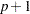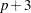The POWER Procedure
 MULTREG Statement

MULTREG <options> ;

The MULTREG statement performs power and sample size analyses for Type IIItests of sets of predictors in multiple linear regression, assuming either fixed or normally distributed predictors.

Summary of Options

Table 68.4 summarizes categories of options available in the MULTREG statement.

Table 68.4 Summary of Options in the MULTREG Statement

Options

Define analysis

Specify analysis information

Specify effects

Specify sample size

Specify power

Control sample size rounding

Control ordering in output

Table 68.5 summarizes the valid result parameters in the MULTREG statement.

Table 68.5 Summary of Result Parameters in the MULTREG Statement

Analyses

Solve For

Syntax

TEST=TYPE3

Power

Sample size

Dictionary of Options

ALPHA=number-list

specifies the level of significance of the statistical test. The default is 0.05, corresponding to the usual 0.05100% = 5% level of significance. See the section Specifying Value Lists in Analysis Statements for information about specifying the number-list.

MODEL=keyword-list

specifies the assumed distribution of the tested predictors. MODEL=FIXED indicates a fixed predictor distribution. MODEL=RANDOM (the default) indicates a joint multivariate normal distribution for the response and tested predictors. You can use the aliases CONDITIONAL for FIXED and UNCONDITIONAL for RANDOM. See the section Specifying Value Lists in Analysis Statements for information about specifying the keyword-list.

FIXED

fixed predictors

RANDOM

random (multivariate normal) predictors

NFRACTIONAL
NFRAC

enables fractional input and output for sample sizes. See the section Sample Size Adjustment Options for information about the ramifications of the presence (and absence) of the NFRACTIONAL option.

NFULLPREDICTORS=number-list
NFULLPRED=number-list

specifies the number of predictors in the full model, not counting the intercept. See the section Specifying Value Lists in Analysis Statements for information about specifying the number-list.

NOINT

specifies a no-intercept model (for both full and reduced models). By default, the intercept is included in the model. If you want to test the intercept, you can specify the NOINT option and simply consider the intercept to be one of the predictors being tested. See the section Specifying Value Lists in Analysis Statements for information about specifying the number-list.

NREDUCEDPREDICTORS=number-list
NREDUCEDPRED=number-list
NREDPRED=number-list

specifies the number of predictors in the reduced model, not counting the intercept. This is the same as the difference between values of the NFULLPREDICTORS= and NTESTPREDICTORS= options. Note that supplying a value of 0 is the same as specifying antest of a Pearson correlation. This option cannot be used at the same time as the NTESTPREDICTORS= option. See the section Specifying Value Lists in Analysis Statements for information about specifying the number-list.

NTESTPREDICTORS=number-list
NTESTPRED=number-list

specifies the number of predictors being tested. This is the same as the difference between values of the NFULLPREDICTORS= and NREDUCEDPREDICTORS= options. Note that supplying identical values for the NTESTPREDICTORS= and NFULLPREDICTORS= options is the same as specifying antest of a Pearson correlation. This option cannot be used at the same time as the NREDUCEDPREDICTORS= option. See the section Specifying Value Lists in Analysis Statements for information about specifying the number-list.

NTOTAL=number-list

specifies the sample size or requests a solution for the sample size with a missing value (NTOTAL=.). The minimum acceptable value for the sample size depends on the MODEL=, NOINT, NFULLPREDICTORS=, NTESTPREDICTORS=, and NREDUCEDPREDICTORS= options. It ranges fromto, whereis the value of the NFULLPREDICTORS option. See Table 68.30 for further information about minimum NTOTAL values, and see the section Specifying Value Lists in Analysis Statements for information about specifying the number-list.

OUTPUTORDER=INTERNAL
OUTPUTORDER=REVERSE
OUTPUTORDER=SYNTAX

controls how the input and default analysis parameters are ordered in the output. OUTPUTORDER=INTERNAL (the default) arranges the parameters in the output according to the following order of their corresponding options:

The OUTPUTORDER=SYNTAX option arranges the parameters in the output in the same order in which their corresponding options are specified in the MULTREG statement. The OUTPUTORDER=REVERSE option arranges the parameters in the output in the reverse of the order in which their corresponding options are specified in the MULTREG statement.

PARTIALCORR=number-list
PCORR=number-list

specifies the partial correlation between the tested predictors and the response, adjusting for any other predictors in the model. See the section Specifying Value Lists in Analysis Statements for information about specifying the number-list.

POWER=number-list

specifies the desired power of the test or requests a solution for the power with a missing value (POWER=.). The power is expressed as a probability, a number between 0 and 1, rather than as a percentage. See the section Specifying Value Lists in Analysis Statements for information about specifying the number-list.

RSQUAREDIFF=number-list
RSQDIFF=number-list

specifies the difference inbetween the full and reduced models. This is equivalent to the proportion of variation explained by the predictors you are testing. It is also equivalent to the squared semipartial correlation of the tested predictors with the response. See the section Specifying Value Lists in Analysis Statements for information about specifying the number-list.

RSQUAREFULL=number-list
RSQFULL=number-list

specifies theof the full model, whereis the proportion of variation explained by the model. See the section Specifying Value Lists in Analysis Statements for information about specifying the number-list.

RSQUAREREDUCED=number-list
RSQREDUCED=number-list
RSQRED=number-list

specifies theof the reduced model, whereis the proportion of variation explained by the model. If the reduced model is an empty or intercept-only model (in other words, if NREDUCEDPREDICTORS=0 or NTESTPREDICTORS=NFULLPREDICTORS), then RSQUAREREDUCED=0 is assumed. See the section Specifying Value Lists in Analysis Statements for information about specifying the number-list.

TEST=TYPE3

specifies a Type IIItest of a set of predictors adjusting for any other predictors in the model. This is the default test option.

Restrictions on Option Combinations

To specify the number of predictors, use any two of these three options:

To specify the effect, choose one of the following parameterizations:

Option Groups for Common Analyses

This section summarizes the syntax for the common analyses supported in the MULTREG statement.

Type III F Test of a Set of Predictors

You can express effects in terms of partial correlation, as in the following statements. Default values of the TEST=, MODEL=, and ALPHA= options specify a Type IIItest with a significance level of 0.05, assuming normally distributed predictors.

```proc power;
multreg
model = random
nfullpredictors = 7
ntestpredictors = 3
partialcorr = 0.35
ntotal = 100
power = .;
run;
```

You can also express effects in terms of:

```proc power;
multreg
model = fixed
nfullpredictors = 7
ntestpredictors = 3
rsquarefull = 0.9
rsquarediff = 0.1
ntotal = .
power = 0.9;
run;
```Previous Page | Next Page | Top of Page